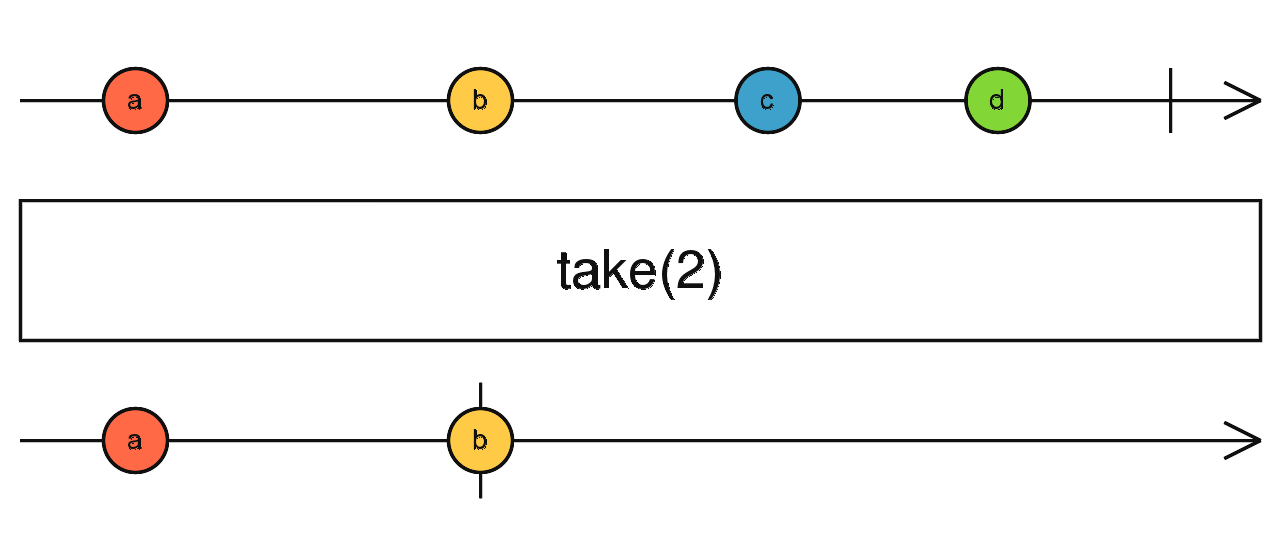/RxJS

# take

`function` `stable`

Emits only the first `count` values emitted by the source Observable.

### `take<T>(count: number): MonoTypeOperatorFunction<T>`

#### Parameters

 count The maximum number of `next` values to emit.

#### Returns

`MonoTypeOperatorFunction<T>`: An Observable that emits only the first `count` values emitted by the source Observable, or all of the values from the source if the source emits fewer than `count` values.

#### Throws

`ArgumentOutOfRangeError` When using `take(i)`, it delivers an ArgumentOutOrRangeError to the Observer's `error` callback if `i < 0`.

## Description

Takes the first `count` values from the source, then completes.`take` returns an Observable that emits only the first `count` values emitted by the source Observable. If the source emits fewer than `count` values then all of its values are emitted. After that, it completes, regardless if the source completes.

## Example

Take the first 5 seconds of an infinite 1-second interval Observable

```import { interval } from 'rxjs';
import { take } from 'rxjs/operators';

const intervalCount = interval(1000);
const takeFive = intervalCount.pipe(take(5));
takeFive.subscribe(x => console.log(x));

// Logs:
// 0
// 1
// 2
// 3
// 4```